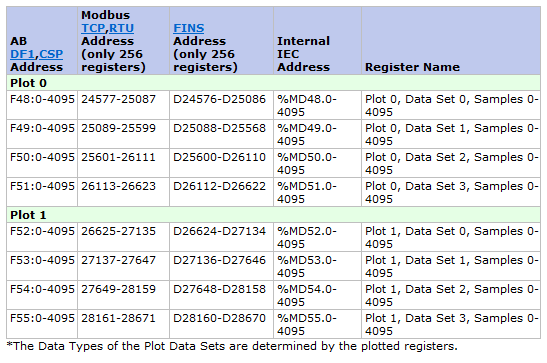# Using Plot Data in User Programs

Captured plot data in the RMC can be read from within user programs by using the REG_REAL, REG_DINT, or REG_DWORD functions. The plot data is not directly accessible via tags and therefore these functions are required. Once you read the plot data, it can be used for calculations, etc. in user programs.

This is an example of how to access RMC75 plot data from a User Program:

1. Declare an array variable large enough for all of the data points you need to capture. In this example there are 20 items.

2. Determine the memory register address for the plot you need to capture items from. The Register Map topic in the Help file will indicate the register number you need. You will be pulling data from the Static Plot Upload Area, which starts at 48 for the RMC75 in this example.3. Use an Expression to read the plot data and write it to the array. If the data is a DINT or DWORD, you will need to replace the REG_REAL function with either REG_DINT or REG_DWORD.